Courses

# Test: Physics - 1

## 20 Questions MCQ Test Science & Technology for UPSC CSE | Test: Physics - 1

Description
This mock test of Test: Physics - 1 for UPSC helps you for every UPSC entrance exam. This contains 20 Multiple Choice Questions for UPSC Test: Physics - 1 (mcq) to study with solutions a complete question bank. The solved questions answers in this Test: Physics - 1 quiz give you a good mix of easy questions and tough questions. UPSC students definitely take this Test: Physics - 1 exercise for a better result in the exam. You can find other Test: Physics - 1 extra questions, long questions & short questions for UPSC on EduRev as well by searching above.
QUESTION: 1

### An iron needle sinks in water whereas a ship made of iron floats on it because

Solution:

Because the specific gravity of the needle is greater than that of water displaced by it. Specific gravity (or relative density) = Density of the substance / Density of the water at 4°C The average density of iron needle is greater than water, therefore, it sinks.

QUESTION: 2

### The cloudy nights are warmer than clear nights because

Solution:

The cloudy nights are warmer because clouds prevent escape of radiation of heat from the ground and the air.

QUESTION: 3

### Food gets cooked faster in a pressure cooker because

Solution:

Because water starts boiling at a lower temperature due to high pressure. According to Gay-Lussac’s law ρ ∝ T.

QUESTION: 4

It is more comfortable to wear white cloths in summer because

Solution:

The white clothes reflect heat falling on them in summer and they absorb the least from the sun.

QUESTION: 5

Of the two bulbs in a house, one glows brighter than the other. Which of the following statements is correct?

Solution:

The dimmer bulb has larger resistance than the brighter one. Power of the bulb ∝ 1 / Resistance Resistance is the obstruction offer to the flow of current.

QUESTION: 6

A fluorescent tube is preferred to an electric bulb because

Solution:

In a fluorescent tube, electrical energy is almost converted into light. The inner wall of the fluorescent tube is coated with phosphorus which immediately transformed into bright light.

QUESTION: 7

Consider the following statements in respect of a jet engine and a rocket:
1. A jet engine uses the surrounding air for its oxygen supply and so is unsuitable for motion in space.
2. A rocket carries its own supply of oxygen in the gas form as a fuel.

Q. Which of the above statement(s) is/are correct?

Solution:

In respect of a jet engine and a rocket, both the given statements are correct.

QUESTION: 8

The leaning tower of Pisa does not fall because

Solution:

Because the vertical line through the centre of gravity of the tower falls within the base.

QUESTION: 9

Electronic motors operating at low voltages tend to burn out because

Solution:

Electronic motors draw more current which is inversely proportional to the voltage, therefore, more heat H = I2Rt is generated.

QUESTION: 10

If the doors of a refrigerator are left open for a few hours, the room temperature will

Solution:

If the doors of a refrigerator are left open for a few hours, the room temperature will increase as the system release heat to the surroundings.

QUESTION: 11

If a rock is brought from the surface of the moon to the earth, then

Solution:

If a rock is brought from the surface of the moon to the earth, its weight will change but not its mass. Mass is a invariant physical quantity whereas weight of a body (w = mg) is variable as the value of acceleration due to gravity (g) changes.

QUESTION: 12

Match List-I with List-II and select the correct answer using the codes given below the lists: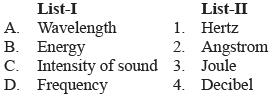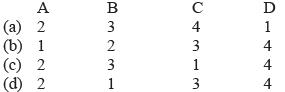Solution:

Wavelength is the distance between two consecutive crests or troughs and 1 angstrom = 10–10m, joule is the unit of energy. Intensity of sound is measured in decibel. Frequency is measured in hertz (Hz).

QUESTION: 13

Match List-I with List-II and select the correct answer with the help of codes given below: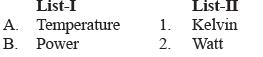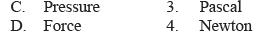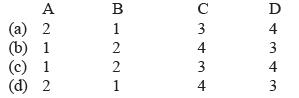Solution:

The unit of temperature is kelvin, power is measured in watt, pressure in pascal and force in newton.

QUESTION: 14

Which one of the following statements is not true about cosmic rays?

Solution:

Cosmic rays have very high frequency >1023 Hz and wavelength <10–24 m.

QUESTION: 15

Pendulum clocks become slow in summer because

Solution:

Pendulum clocks become slow in summer because the length of the pendulum increases. Increase in length ∆l = lo α ∆T where lo = original length, α = coefficient of linear expansion and ∆T = change in temperature.

QUESTION: 16

Consider the following statements and select the correct code:
Assertion (A): A piece of ice added to the drink cools it.
Reason (R): Ice takes latent heat from the drink for melting resulting in the cooling of the drink.

Solution:

A piece of ice added to the drink cool it because ice takes latent heat from the drink for melting resulting in the cooling of the drink.

QUESTION: 17

Match List-I with List-II and select the correct answer using the codes given below the lists: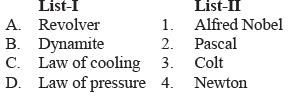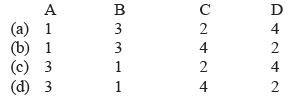Solution:

Revolver was discovered by Colt. Dynamite was discovered by Alfred Nobel. Law of cooling was discovered by Newton and law of pressure by Pascal.

QUESTION: 18

The atmosphere exerts enormous pressure on us. But, we don’t feel it because

Solution:

We don’t feel the atmospheric pressure because our blood exerts a pressure slightly more than that of the atmosphere.

QUESTION: 19

Which one of the following statements is not true for a person suffering from hypermetropia?

Solution:

Hypermetropia is corrected by using a convex lens.

Myopia is corrected by concave lens.

QUESTION: 20

Consider the following statements and select the correct answer using the codes given below:
Assertion (A): If ice collects on the freezer, the cooling in the refrigerator is affected adversely.
Reason (R): Ice is a poor conductor.

Solution:

If ice collects on the freezer, the cooling in the refrigerator is affected adversely because ice is a poor conductor of heat.